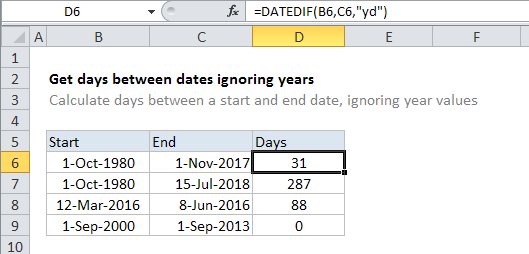# Get days between dates ignoring years in Excel

To calculate days between two dates, ignoring year values, use the DATEDIF function.

## Formula

`=DATEDIF(start_date,end_date,"yd")`In the example shown, the formula in D6 is:

`=DATEDIF(B6,C6,"yd")`

## Explanation

The DATEDIF function can handle a variety of “date difference” calculations to calculate the difference between two dates in years, months, and days.  DATEDIF takes 3 arguments: start date, end_date, and “unit”, which controls which result is returned.

Also See:   How to get number of days, weeks, months or years between two dates in Excel

In this case, we want days ignoring years so we supply “yd” for unit. (For the full list of options, see the DATEDIF page).

Once configured, the function is fully automatic and returns a result in the unit requested.